Éducation par Wolfram Group LLC

# Wolfram Calculus Course Assistant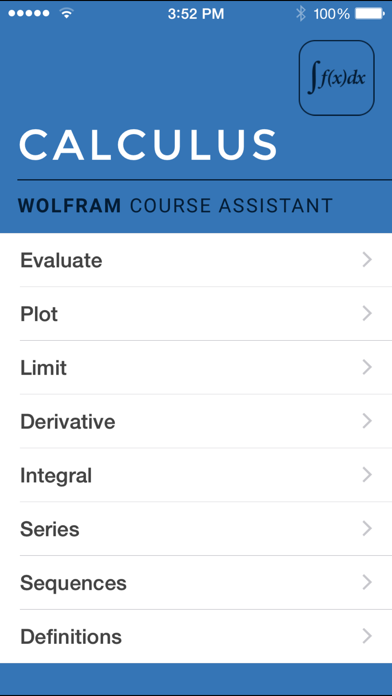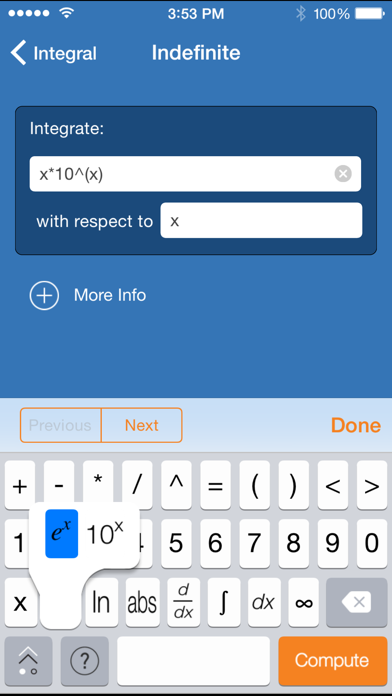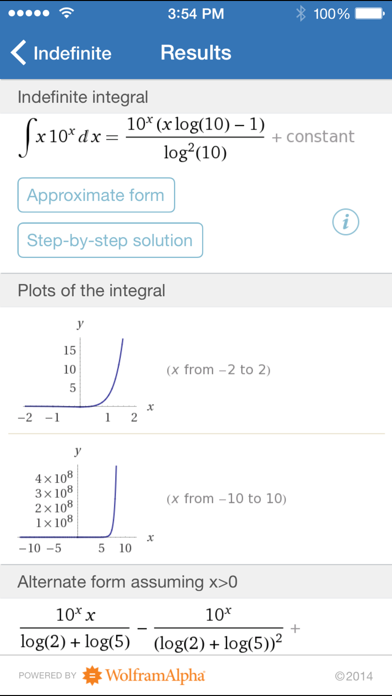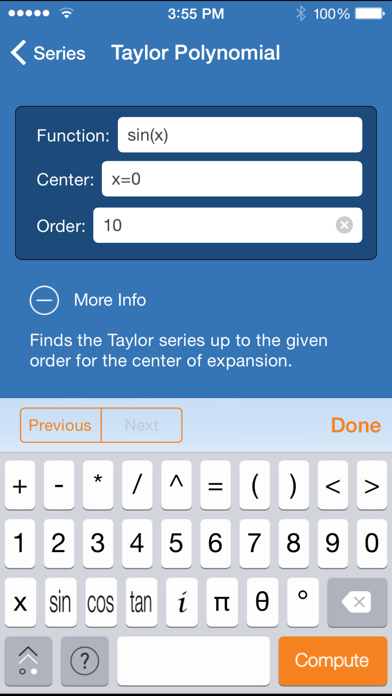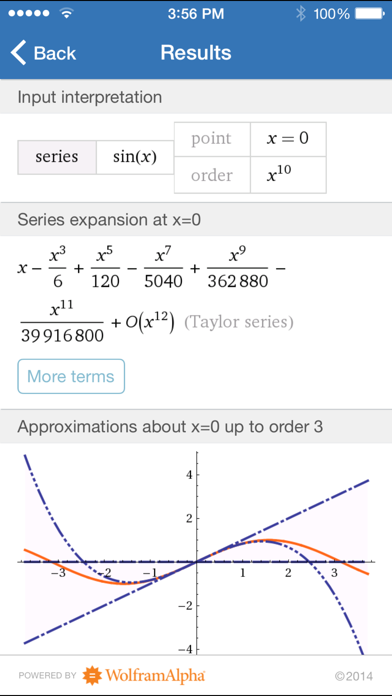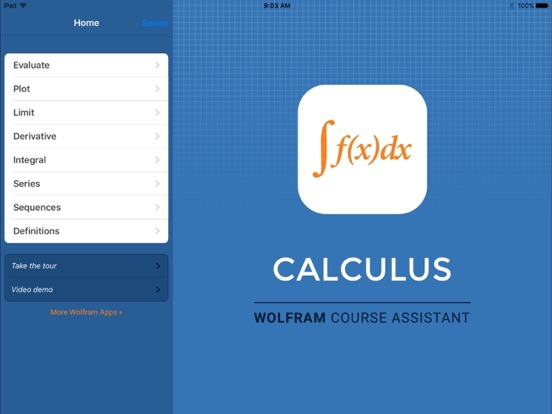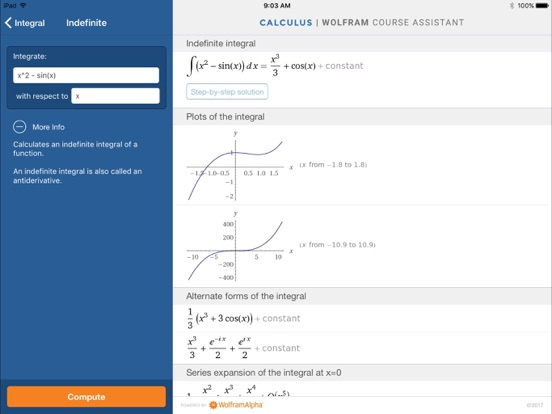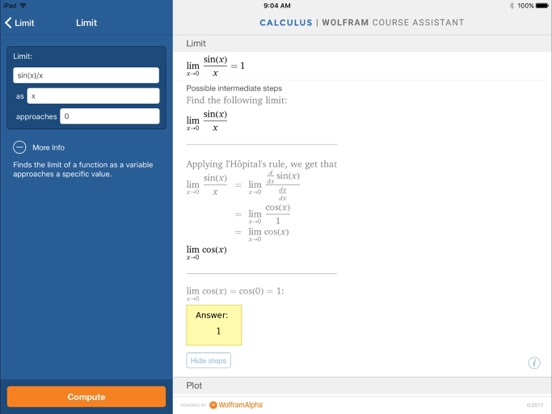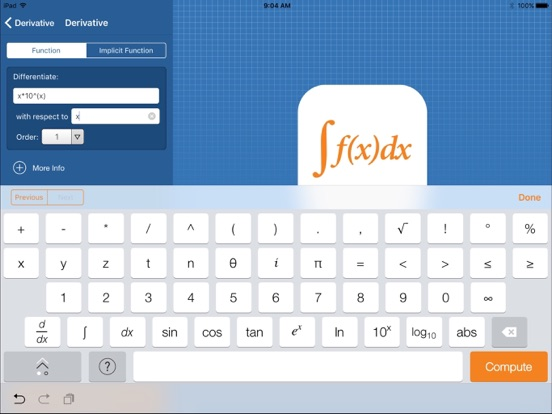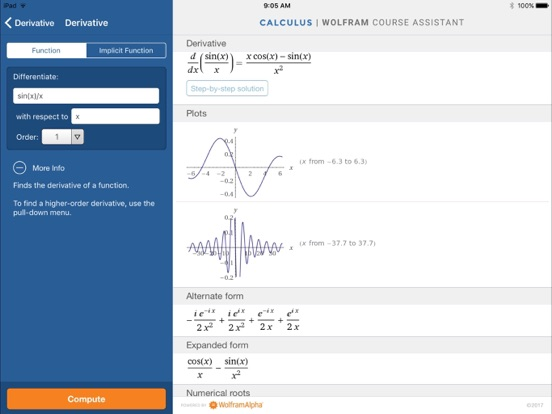⭐⭐⭐⭐★ (3 votes, moyenne : 4,00 / 5)

Version : 1.3.1

Compatibilité : iOS 9.3 +

Taille : 14,67 Mo

Prix : 3,99 €##### Description
Taking calculus? Then you need the Wolfram Calculus Course Assistant. This definitive app for calculus—from the world leader in math software—will help you work through your homework problems, ace your tests, and learn calculus concepts. Forget canned examples! The Wolfram Calculus Course Assistant solves your specific Calculus problems on the fly including step-by-step guidance for derivatives, integrals, and much more.

This app covers the following topics applicable to Calculus, AP Calculus AB, AP Calculus BC, Calculus I, and Calculus II:

– Evaluate any numeric expression or substitute a value for a variable.
– Plot basic, parametric, or polar plots of the function(s) of your choice.
– Determine the limit of a function as it approaches a specific value.
– Differentiate any function or implicit function.
– Find the critical points and inflection points of a function.
– Identify the local and absolute extrema of a function.
– Integrate a function, with or without limits.
– Sum a function given a lower and upper bound.
– Find the closed form of a sequence or generate terms for a specific sequence.

The Wolfram Calculus Course Assistant is powered by the Wolfram|Alpha computational knowledge engine and is created by Wolfram Research, makers of Mathematica—the world’s leading software system for mathematical research and education.

The Wolfram Calculus Course Assistant draws on the computational power of Wolfram|Alpha’s supercomputers over a 3G, 4G, or Wi-Fi connection.

Version 1.3.1

##### Changements récents
This app has been updated by Apple to display the Apple Watch app icon.

– Bug fixes